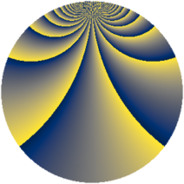# Properties

 Label 2736.2.hjLevel $2736$ Weight $2$ Character orbit 2736.hj Rep. character $\chi_{2736}(275,\cdot)$ Character field $\Q(\zeta_{36})$ Dimension $5712$ Sturm bound $960$

# Related objects

## Defining parameters

 Level: $$N$$ $$=$$ $$2736 = 2^{4} \cdot 3^{2} \cdot 19$$ Weight: $$k$$ $$=$$ $$2$$ Character orbit: $$[\chi]$$ $$=$$ 2736.hj (of order $$36$$ and degree $$12$$) Character conductor: $$\operatorname{cond}(\chi)$$ $$=$$ $$2736$$ Character field: $$\Q(\zeta_{36})$$ Sturm bound: $$960$$

## Dimensions

The following table gives the dimensions of various subspaces of $$M_{2}(2736, [\chi])$$.

Total New Old
Modular forms 5808 5808 0
Cusp forms 5712 5712 0
Eisenstein series 96 96 0

## Trace form

 $$5712q - 18q^{2} - 12q^{3} - 6q^{4} - 18q^{5} - 12q^{6} - 24q^{7} + O(q^{10})$$ $$5712q - 18q^{2} - 12q^{3} - 6q^{4} - 18q^{5} - 12q^{6} - 24q^{7} - 24q^{10} - 18q^{11} - 6q^{12} - 6q^{13} - 18q^{14} - 6q^{16} - 24q^{18} - 24q^{19} - 36q^{20} - 48q^{21} - 6q^{22} - 36q^{23} - 12q^{24} - 144q^{26} - 6q^{27} - 48q^{28} - 18q^{29} + 36q^{30} - 18q^{32} - 24q^{33} - 6q^{34} - 90q^{35} - 12q^{36} - 48q^{37} - 18q^{38} - 48q^{39} - 6q^{40} - 24q^{42} - 6q^{43} + 198q^{44} - 6q^{45} - 12q^{46} - 12q^{48} + 5400q^{49} - 18q^{50} - 12q^{51} - 6q^{52} - 144q^{54} - 48q^{55} - 162q^{56} - 12q^{58} - 18q^{59} + 30q^{60} - 6q^{61} - 36q^{62} - 12q^{64} - 36q^{65} + 48q^{66} - 6q^{67} - 6q^{69} - 48q^{70} - 48q^{72} - 18q^{74} + 144q^{75} - 6q^{76} - 36q^{77} - 24q^{78} - 24q^{81} - 24q^{82} - 174q^{84} - 6q^{85} - 18q^{86} - 12q^{87} - 12q^{88} - 228q^{90} - 66q^{91} - 18q^{92} - 30q^{93} - 12q^{94} - 174q^{96} - 12q^{97} - 162q^{98} + 42q^{99} + O(q^{100})$$

## Decomposition of $$S_{2}^{\mathrm{new}}(2736, [\chi])$$ into newform subspaces

The newforms in this space have not yet been added to the LMFDB.